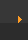## Stoichiometry: Molecules to Moles

 To convert between moles and molecules you need to remember that one mole of any substance contains 6.02 x 1023 particles (e.g., atoms or molecules). When going from moles to molecules you multiply by 6.02 x 1023. When going from molecules to moles you divide by 6.02 x 1023. Convert 4.0 moles to molecules. Convert 8.4 x 1023 molecules to moles. Convert 37.8 mole of O2 to molecules. Convert 3.72 x 109 molecules to moles. Reset this page The mole is a frequently used unit for the amount of atoms, molecules, ions, etc. One mole of any element consists of 6.02 x 1023 particles (e.g., atoms or molecules). 6.02 x 1023 is known as Avogadro's Number. The mass of one mole of an element is called the Gram Atomic Mass (GAM). For compounds it's called the Gram Formula Mass (GFM). The periodic table lists the mass of one mole or 6.02 x 1023 atoms of each element (e.g. one mole of H is 1.0079 g).